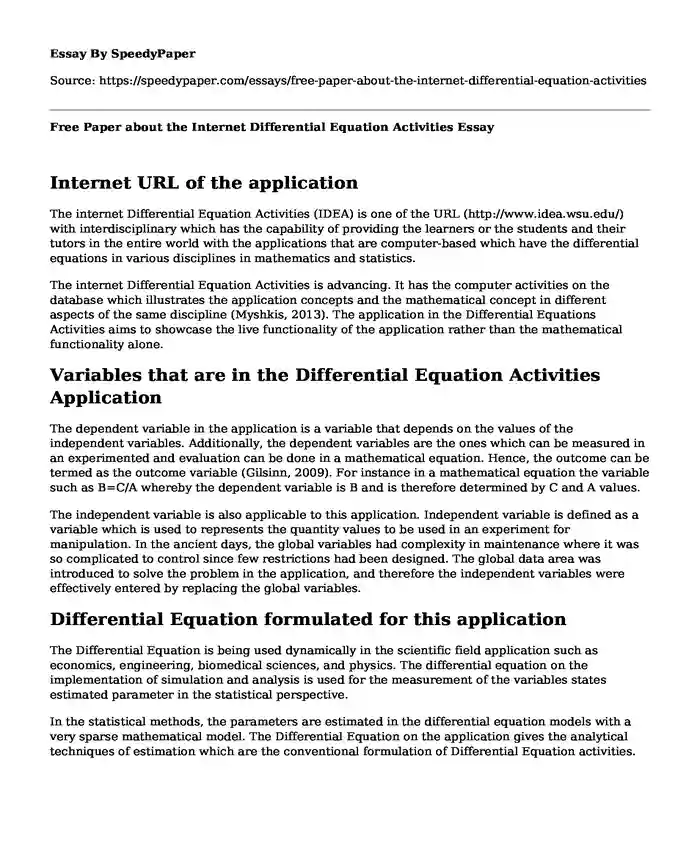# Free Paper about the Internet Differential Equation Activities

Published: 2022-09-28Type of paper: Research paper Categories: Mathematics Pages: 3 Wordcount: 594 words
143 views

## Internet URL of the application

The internet Differential Equation Activities (IDEA) is one of the URL (http://www.idea.wsu.edu/) with interdisciplinary which has the capability of providing the learners or the students and their tutors in the entire world with the applications that are computer-based which have the differential equations in various disciplines in mathematics and statistics.Is your time best spent reading someone else’s essay? Get a 100% original essay FROM A CERTIFIED WRITER!

The internet Differential Equation Activities is advancing. It has the computer activities on the database which illustrates the application concepts and the mathematical concept in different aspects of the same discipline (Myshkis, 2013). The application in the Differential Equations Activities aims to showcase the live functionality of the application rather than the mathematical functionality alone.

## Variables that are in the Differential Equation Activities Application

The dependent variable in the application is a variable that depends on the values of the independent variables. Additionally, the dependent variables are the ones which can be measured in an experimented and evaluation can be done in a mathematical equation. Hence, the outcome can be termed as the outcome variable (Gilsinn, 2009). For instance in a mathematical equation the variable such as B=C/A whereby the dependent variable is B and is therefore determined by C and A values.

The independent variable is also applicable to this application. Independent variable is defined as a variable which is used to represents the quantity values to be used in an experiment for manipulation. In the ancient days, the global variables had complexity in maintenance where it was so complicated to control since few restrictions had been designed. The global data area was introduced to solve the problem in the application, and therefore the independent variables were effectively entered by replacing the global variables.

## Differential Equation formulated for this application

The Differential Equation is being used dynamically in the scientific field application such as economics, engineering, biomedical sciences, and physics. The differential equation on the implementation of simulation and analysis is used for the measurement of the variables states estimated parameter in the statistical perspective.

In the statistical methods, the parameters are estimated in the differential equation models with a very sparse mathematical model. The Differential Equation on the application gives the analytical techniques of estimation which are the conventional formulation of Differential Equation activities.

## The useful technique used to solve Differential Equation

The Differential Equation was solved using the separable differential equations. This technique uses the separating variables which are non -linear in potential (Balachandran, 2009). The separable uses this form M(x) +N(y) dydx=0. The integration is therefore done respectively to the variable M which is respect to variable x and in respect to variable N which is respect to variable y too. The separable technique isolates the equations on the sides and another variable on the other sides and hence integrating the equation. This technique of separable equation results in finding the implicit solution for the Differential Equations.

## The exciting aspect of the Application

The interesting issue of the application is that it focuses on bringing experts on the field to strengthen various areas of the Differential Equation (Kolmanovskii, 2013). It majorly deals with the probability modeling of the applications processes and the classical analysis in the engineering and mathematical. The application promotes the interaction on the particular focus for modeling issues in the mathematical aspects and the derivations in the numerical analysis solution.

Works Cited

Balachandran, Balakumar, Tamas Kalmar-Nagy, and David E. Gilsinn. Delay differential equations. Berlin: Springer, 2009.

Kolmanovskii, V., and A. Myshkis. Introduction to the theory and applications of functional differential equations. Vol. 463. Springer Science & Business Media, 2013.